Version:

This documentation is not for the latest stable Salvus version.This tutorial is presented as Python code running inside a Jupyter Notebook, the recommended way to use Salvus. To run it yourself you can copy/type each individual cell or directly download the full notebook, including all required files.# 2D Topography and Vertical Refinements

## Beyond piecewise structured grids

### Vertical refinement in layers with strong thickness variation

This notebooks shows how problems with small time steps in layers with strongly varying thickness can be avoided. One prominent example is the crustal thickness variations.

Salvus provides structured grid classes in 2D and 3D, that allow to vary the number of elements in one dimension, creating anisotropic element sizes. When combined with a corresponding topography model, the elements end up with isotropic sizes again and avoid the small time step that would result from a regular grid.

The workflow is as follows:

• create a topography model
• compute the number of elements needed in vertical direction according to the resolution criterion
• create a structured grid with anisotropic refinement
• convert to an unstructured mesh and deform the elements according to the topography
Copy
# initialize notebook
%matplotlib inline

import matplotlib.pyplot as plt
import numpy as np

plt.rcParams["figure.figsize"] = (12, 8)
from salvus.mesh.structured_grid_2D import StructuredGrid2D
from salvus.mesh.skeleton import Skeleton

# general input
max_x = 10000.0  # domain width in m
fmax = 2.0  # maximum frequency in Hz
elements_per_wavelength = 2.0
vs = 1000.0  # s-wave velocity in m / s
vp = 1700.0  # p-wave velocity in m / s

refine_x = 1.2  # extra security factor to make elements smaller before
refine_y = 1.2  # deformation

# compute edgelengths
hmax = vs / fmax / elements_per_wavelength

# create some artificial topography model - h(x)
nelem_x = int(np.ceil(max_x / hmax * refine_x))
h0 = 0.6
h1 = 0.5
h2 = -0.1
h3 = 0.15

x = np.linspace(0.0, max_x, nelem_x + 1)
norm_x = x / max_x * 2 * np.pi
h = (
h0
- h1 * np.cos(norm_x)
- h2 * np.cos(2 * norm_x)
- h3 * np.sin(3 * norm_x)
)
h = h * max_x / 2 / np.pi

# number of vertical elements needed for each element in horizontal direction
nelem_y = np.ceil(h / hmax * refine_y).astype("int")

# create box mesh with refinements
sg1 = StructuredGrid2D.rectangle_vertical_refine_doubling(
nelem_x, nelem_y, min_x=0, max_x=max_x, min_y=0.0, max_y=np.max(h)
)

# make unstructured mesh
m1 = sg1.get_unstructured_mesh()
m1.find_side_sets("cartesian")
m1.plot(linewidths=1.0)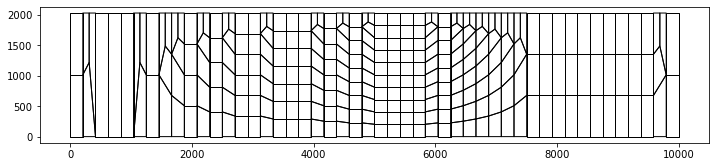At this point we have a somewhat unusal looking mesh on a cartesian domain and varying number of elements in vertical direction. Once we deform it according to the topography, it looks much more reasonable

m1.add_dem_2D(x, h - np.max(h))
m1.apply_dem()
m1.plot(linewidths=1.0)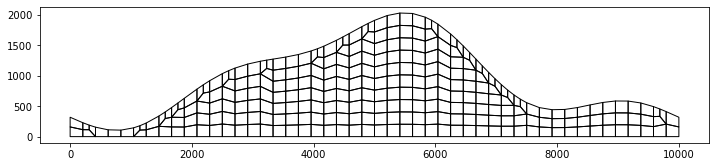For reference we also create a mesh without refinements,

sg2 = StructuredGrid2D.rectangle(
nelem_x, max(nelem_y), min_x=0, max_x=max_x, min_y=0.0, max_y=np.max(h)
)
m2 = sg2.get_unstructured_mesh()
m2.find_side_sets("cartesian")
m2.plot(linewidths=1.0)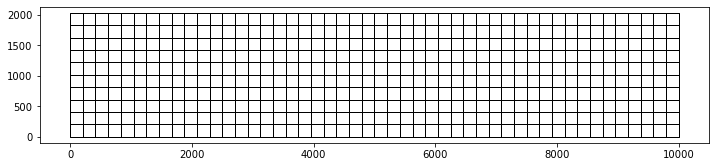which has very thin elements after applying topography:

m2.add_dem_2D(x, h - np.max(h))
m2.apply_dem()
m2.plot(linewidths=1.0)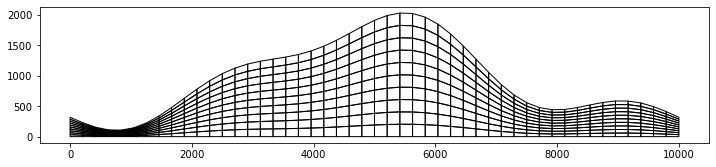These result in small time steps due to the Courant criterion discussed in exercise 2. We again use the accurate algorithm to estimate $\Delta t$, which accounts for the shape of the elements:

dt1, dt1_elem = m1.compute_dt(vp, fast=False)
dt2, dt2_elem = m2.compute_dt(vp, fast=False)

print("dt1:       {:10.4f}".format(dt1))
print("dt2:       {:10.4f}".format(dt2))
print("dt1 / dt2: {:10.4f}".format(dt1 / dt2))

# plot dt over the mesh
m1.attach_field("dt", dt1_elem)
m2.attach_field("dt", dt2_elem)
dt1:           0.0199
dt2:           0.0061
dt1 / dt2:     3.2646


If we include the number of elements, which is also reduced with the anistropic refinement, we can estimate the resulting speed up of the simulation:

cost1 = m1.nelem / dt1
cost2 = m2.nelem / dt2

print("nelem1:  ", m1.nelem)
print("nelem2:  ", m2.nelem)
print("speedup:  {:.2f}".format(cost2 / cost1))
nelem1:   273
nelem2:   480
speedup:  5.74

m1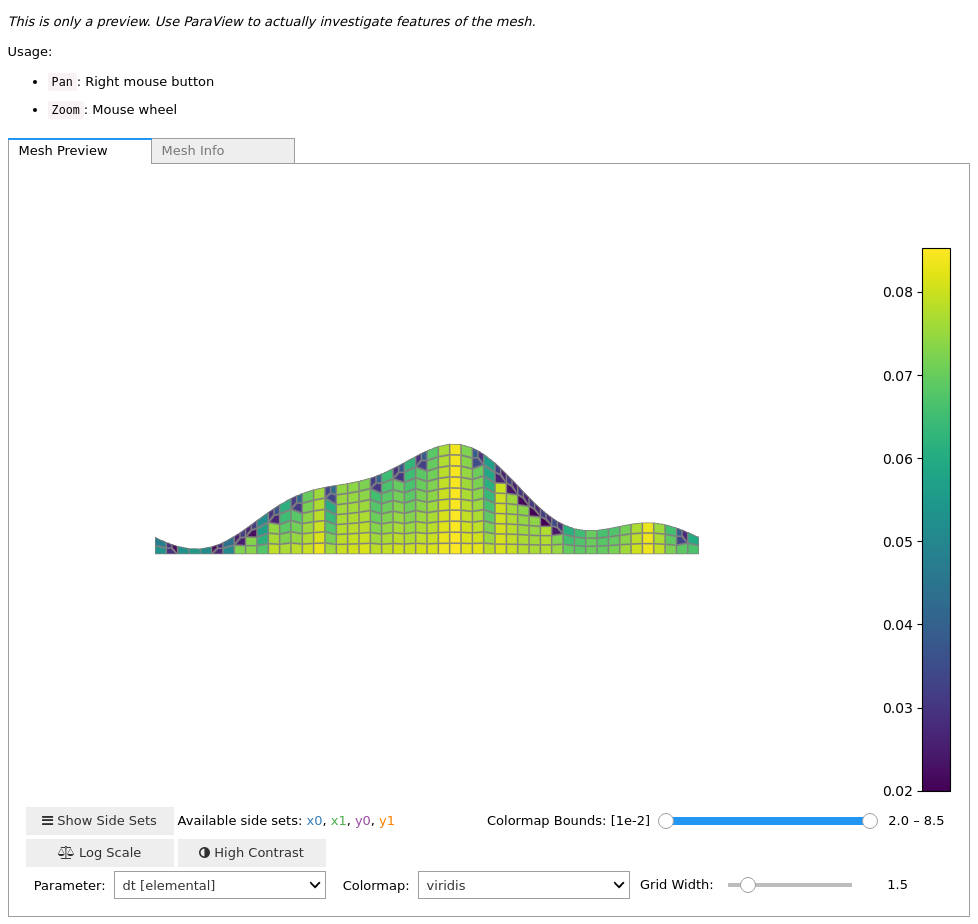<salvus.mesh.unstructured_mesh.UnstructuredMesh at 0x7f3304139710>
m2